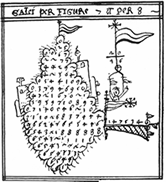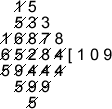#### You may also like### More Mods

What is the units digit for the number 123^(456) ?### Expenses

What is the largest number which, when divided into 1905, 2587, 3951, 7020 and 8725 in turn, leaves the same remainder each time?### Factorial

How many zeros are there at the end of the number which is the product of first hundred positive integers?

# Galley Division

##### Age 14 to 16 Challenge Level:The 'Galley' method of division was probably the most widely used method of division prior to the 1600s. It is called 'Galley' because, when completed, a division looks a bit like a galley (boat).

The Galley method is thought to be Hindu in origin and an early version of this method was known to be used in the ninth century by a Persian called Al-Khowarizmi. It is still taught in parts of North Africa and the Middle East today.

This is how you could work out $65284$ divided by $594$ using the Galley method:Can you see the two numbers I started with?
The calculation tells me that $65284 \div 594 = 109$ remainder $538$.
Can you see the answer and the remainder?

Here's another division done using the Galley method:What division do you think is being done here?
What is the answer to this division?

Here are two video clips of divisions using Galley arithmetic:

If you are struggling to see what is happening, try the division using your own method and see if you can recognise numbers that are appearing in the galley method that are also in your own recording. If you need further guidance, there is a division, showing each of the main steps and some explanation, in the hints section of this problem.

Send us answers to the following divisions showing that you have used the same method. $$2986 \div 47$$
$$76254 \div 235$$

Why does the method work?
How does this method compare with our modern methods of division?